## 17: Decision Trees

### Motivation for Decision Trees

Often you don't care about the exact nearest neighbor, you just want to make a prediction. Nearest neighbor search is slow and requires a lot of storage $O(nd)$.
New idea:
1. Build a KD-type tree with only pure leaves
2. Descent test point and make decision based on leaf label. Exact nearest neighbor is not really needed.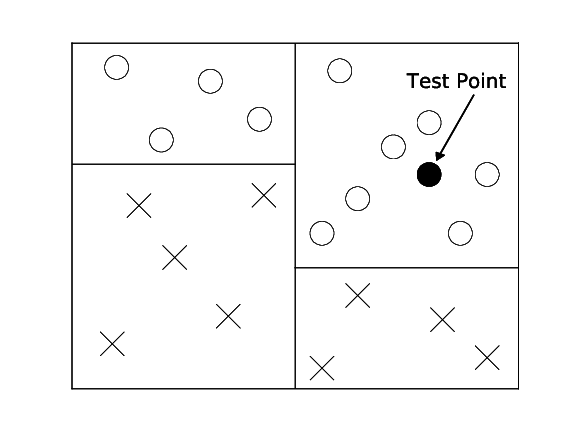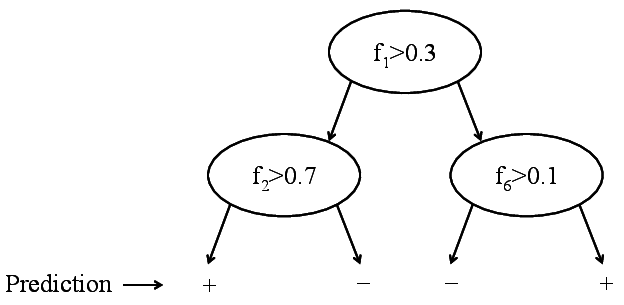Binary decision tree. Only labels are stored.

New goal: Build a tree that is:
1. Maximally compact
2. Only has pure leaves
Quiz: Is it always possible to find a consistent tree?
Yes, if and only if no two input vectors have identical features but different labels
Bad News! Finding a minimum size tree is NP-Hard!!
Good News: We can approximate it very effectively with a greedy strategy. We keep splitting the data to minimize an impurity function that measures label purity amongst the children.

### Impurity Functions

Data: $S=\left \{ \left ( \mathbf{x}_1,y_1 \right ),\dots,\left ( \mathbf{x}_n,y_n \right ) \right \}, y_i\in\left \{ 1,\dots,c \right \}$, where $c$ is the number of classes

#### Gini impurity

Let $S_k\subseteq S$ where $S_k=\left \{ \left ( \mathbf{x},y \right )\in S:y=k \right \}$ (all inputs with labels $k$)
$S=S_1\cup \dots \cup S_c$
Define: $p_k=\frac{\left | S_k \right |}{\left | S \right |}\leftarrow \textrm{fraction of inputs in } S \textrm{ with label } k$
Note: This is different from Gini coefficient. See Gini impurity (not to be confused with the Gini Coefficient) of a leaf: $G(S)=\sum_{k=1}^{c}p_k(1-p_k)$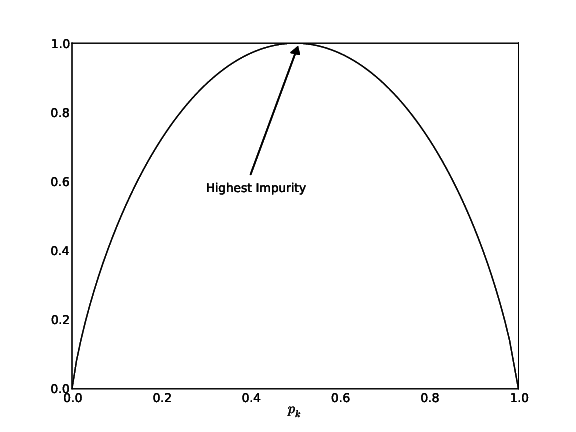Fig: Gini Impurity Function

Gini impurity of a tree: $G^T(S)=\frac{\left | S_L \right |}{\left | S \right |}G^T(S_L)+\frac{\left | S_R \right |}{\left | S \right |}G^T(S_R)$ where:
• $\left ( S=S_L\cup S_R \right )$
• $S_L\cap S_R=\varnothing$
• $\frac{\left | S_L \right |}{\left | S \right |}\leftarrow \textrm{fraction of inputs in left substree}$
• $\frac{\left | S_R \right |}{\left | S \right |}\leftarrow \textrm{fraction of inputs in right substree}$

#### Entropy

Let $p_1,\dots,p_k$ be defined as before. We know what we don't want (Uniform Distribution): $p_1=p_2=\dots=p_c=\frac{1}{c}$ This is the worst case since each leaf is equally likely. Prediction is random guessing. Define the impurity as how close we are to uniform. Use $KL$-Divergence to compute "closeness"
Note: $KL$-Divergence is not a metric because it is not symmetric, i.e., $KL(p||q)\neq KL(q||p)$.
Let $q_1,\dots,q_c$ be the uniform label/distribution. i.e. $q_k=\frac{1}{c} \forall k$ $KL(p||q)=\sum_{k=1}^{c}p_klog\frac{p_k}{q_k}\geq 0 \leftarrow \textrm{KL-Divergence}$ $=\sum_{k}p_klog(p_k)-p_klog(q_k)\textrm{ where }q_k=\frac{1}{c}$ $=\sum_{k}p_klog(p_k)+p_klog(c)$ $=\sum_{k}p_klog(p_k)+log(c)\sum_{k}p_k \textrm{ where } log(c)\leftarrow\textrm{constant}, \sum_{k}p_k=1$
$\max_{p}KL(p||q)=\max_{p}\sum_{k}p_klog(p_k)$ $=\min_{p}-\sum_{k}p_klog(p_k)$ $=\min_{p}H(s) \leftarrow\textrm{Entropy}$
Entropy over tree: $H(S)=p^LH(S^L)+p^RH(S^R)$ $p^L=\frac{|S^L|}{|S|}, p^R=\frac{|S^R|}{|S|}$

### ID3-Algorithm

Base Cases: $\textrm{ID3}(S):\left\{ \begin{array}{ll} \textrm{if } \exists \bar{y}\textrm{ s.t. }\forall(x,y)\in S, y=\bar{y}\Rightarrow \textrm{return leaf } \textrm{ with label } \bar{ y}\\ \textrm{if } \exists\bar{x}\textrm{ s.t. }\forall(x,y)\in S, x=\bar{x}\Rightarrow \textrm{return leaf } \textrm{ with mode}(y:(x,y)\in S)\textrm{ or mean (regression)}\end{array} \right.$ The Equation above indicates the ID3 algorithm stop under two cases. The first case is that all the data points in a subset of have the same label. If this happens, we should stop splitting the subset and create a leaf with label $y$. The other case is there are no more attributes could be used to split the subset. Then we create a leaf and label it with the most common $y$.

Try all features and all possible splits. Pick the split that minimizes impurity $(\textrm{e.g. } s>t)$ where $f\leftarrow$feature and $t\leftarrow$threshold
Recursion: $\textrm{Define: }\begin{bmatrix} S^L=\left \{ (x,y)\in S: x_f\leq t \right \}\\ S^R=\left \{ (x,y)\in S: x_f> t \right \} \end{bmatrix}$
Quiz: Why don't we stop if no split can improve impurity?
Example: XOR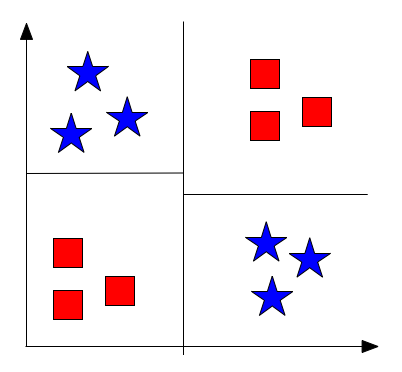Fig 4: Example XOR

• First split does not improve impurity
• Decision trees are myopic

### Regression Trees

#### CART: Classification and Regression Trees

Assume labels are continuous: $y_i\in\mathbb{R}$
Impurity: Squared Loss $L(S)=\frac{1}{|S|}\sum_{(x,y)\in S}(y-\bar{y}_S)^2 \leftarrow\textrm{Average squared difference from average label}$ $\textrm{where }\bar{y}_S=\frac{1}{|S|}\sum_{(x,y)\in S}y\leftarrow\textrm{Average label}$ At leaves, predict $\bar{y}_S$. Finding best split only costs $O(nlogn)$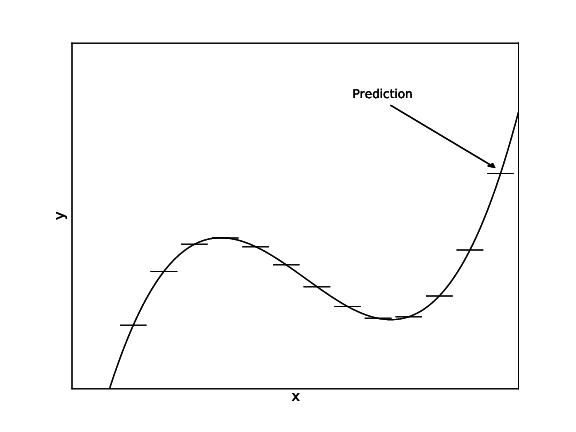Fig: CART

CART summary:
• CART are very light weight classifiers
• Very fast during testing
• Usually not competitive in accuracy but can become very strong through bagging (Random Forests) and boosting (Gradient Boosted Trees)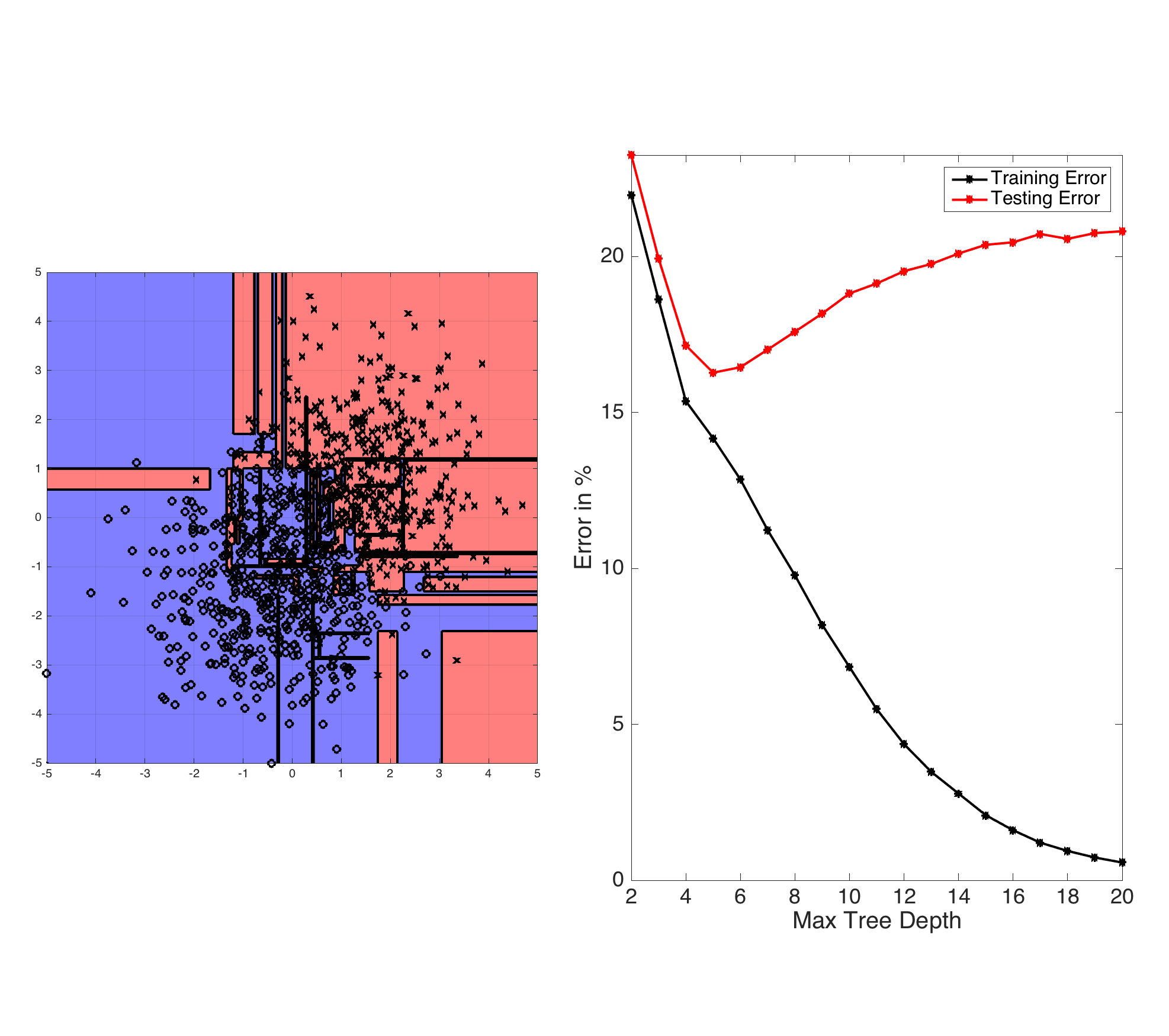Fig: ID3-trees are prone to overfitting as the tree depth increases. The left plot shows the learned decision boundary of a binary data set drawn from two Gaussian distributions. The right plot shows the testing and training errors with increasing tree depth.